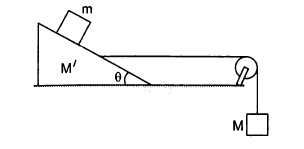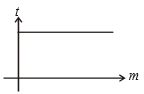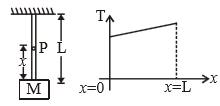A simple pendulum hangs from the roof of a train moving on horizontal rails. If the string is inclined towards the front of the train, then train is

1. Moving with constant velocity

2. In accelerated motion

3. In retarded motion

4. At rest

Concept Questions :-

Newton laws
High Yielding Test Series + Question Bank - NEET 2020

Difficulty Level:

The value of M of the hanging block is in the figure, which will prevent the smaller block (m=1 kg) from slipping over the triangular block. All the surfaces are smooth and string and pulley are ideal. (Given: M'=4 kg and $\theta ={37}^{0}$)1. 12 kg

2. 15 kg

3. 10 kg

4. 4 kg

Concept Questions :-

Pseudo force
High Yielding Test Series + Question Bank - NEET 2020

Difficulty Level:

A lighter body and heavier body both are moving with same momentum and applying same retarding force. Their stoping distance are  respectively. Then the correct relation between .

(1) ${s}_{1}>{s}_{2}$

(2) ${s}_{1}<{s}_{2}$

(3) ${s}_{1}={s}_{2}$

(4) None of these

Concept Questions :-

Newton laws
High Yielding Test Series + Question Bank - NEET 2020

Difficulty Level:

A plastic box of mass 5 kg is found to accelerate up at the rate of g / 6, when placed deep inside the water. How much sand should be put inside the box so that it may accelerate down at the rate of g / 6?

1.  2kg

2.  3kg

3.  4kg

4.  5kg

Concept Questions :-

Application of laws
High Yielding Test Series + Question Bank - NEET 2020

Difficulty Level:

Let $\theta$ denote the angular displacement of a simple pendulum oscillating in a vertical plane. If the mass of bob is m. The tension in the string is mg cos $\theta$

1.  always

2.  never

3.  at extreme

4.  at mean position

Concept Questions :-

Non-Uniform vertical circular motion
High Yielding Test Series + Question Bank - NEET 2020

Difficulty Level:

In a uniform circular motion, which of the following quantity is not constant

(1) Angular momentum

(2) Speed

(3) Kinetic energy

(4) Momentum

Concept Questions :-

Uniform circular motion
High Yielding Test Series + Question Bank - NEET 2020

Difficulty Level:

The graph for stopping time (t) versus mass (m) is shown in the figure. Then which one of the pair is given1.  Momentum, force

2.  K.E., force

3.  Velocity, force

4.  None of these

Concept Questions :-

Application of laws
High Yielding Test Series + Question Bank - NEET 2020

Difficulty Level:

A metal ring of mass m and radius R is placed on smooth horizontal table and is set rotating about its own axis in such a way that each part of the ring moves with a speed v. Tension in the ring is:

(1) $\frac{m{v}^{2}}{R}$

(2) $\frac{m{v}^{2}}{2R}$

(3) $\frac{m{v}^{2}}{2\mathrm{\pi }R}$

(4) None of these

Concept Questions :-

Uniform circular motion
High Yielding Test Series + Question Bank - NEET 2020

Difficulty Level:

Tension on the string at point P is T. The graph for tension (T) versus x is shown in the figure. Then the string is:1.  massless

2.  massfull

3.  tension on every point on the string is same when the string is having finite mass 4

4.  None of these

Concept Questions :-

Application of laws
High Yielding Test Series + Question Bank - NEET 2020

Difficulty Level:

While walking on ice one should take small steps to avoid slipping. This is because smaller steps ensure

(1) Larger friction

(2) Smaller friction

(3) Larger normal force

(4) Smaller normal force

Concept Questions :-

Newton laws